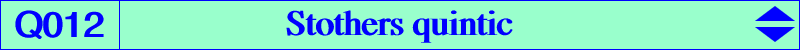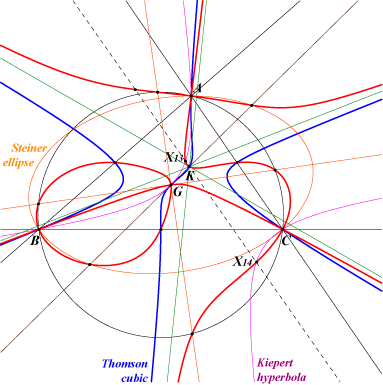∑ [a^2 (y - z) - (b^2 - c^2) x] y^2 z^2 = 0 ∑ a^2 y z (y - z) (x^2 - y z) = 0X(2), X(6), X(7), X(13), X(14), X(673), X(694), X(6189), X(6190) extraversions of X(7), X(673), X(694) points at infinity of the Steiner ellipse and the Thomson cubic midpoints of ABC with tangents passing through K intersections of the axes of the Steiner ellipse and the circumcircle X(6189), X(6190) : intersections of the Steiner ellipse and the line GK 6 points on the symmedians: (±bc,b^2,c^2), (a^2,±ac,c^2), (a^2,b^2,±ab)Q012 is a circum-quintic with four nodes namely A, B, C, G. The nodal tangents at A, B, C are the corresponding internal and external bisectors. The nodal tangents at G are the axes of the Steiner ellipse. Q012 has three real asymptotes parallel to those of the Thomson cubic and concurring at K. It has also two imaginary asymptotes secant at X(599). Locus properties : locus of F such that the Stothers cubic ST(F) has perpendicular nodal tangents. See CL030. locus of point M such that the circum-conic and inconic with same perspector M have parallel axes, or equivalently such that the pencil of conics generated by these two conics contains a circle. See K002, property 22 for related topics. locus of node N of nodal circum-cubics passing through G and having asymptotes parallel to those of the Thomson cubic K002. See K281, K295 for instance. see CL040 for another property. let M1 and M2 be the brocardians of a point M. The perpendicular bisector of M1M2 passes through M if and  only if M lies on Q012 (Angel Montesdeoca, 2022-10-11). Recall that the brocardians of M = (u : v :w) are M1 = (u w : u v : v w) = (1/v : 1/w : 1/u) and M2 = (u v : v w : u w) = (1/w : 1/u : 1/v). See a generalization in Table 28 : cevian and anticevian points and also Cax(F) in CL047. The isogonal transform of Q012 is Q090.